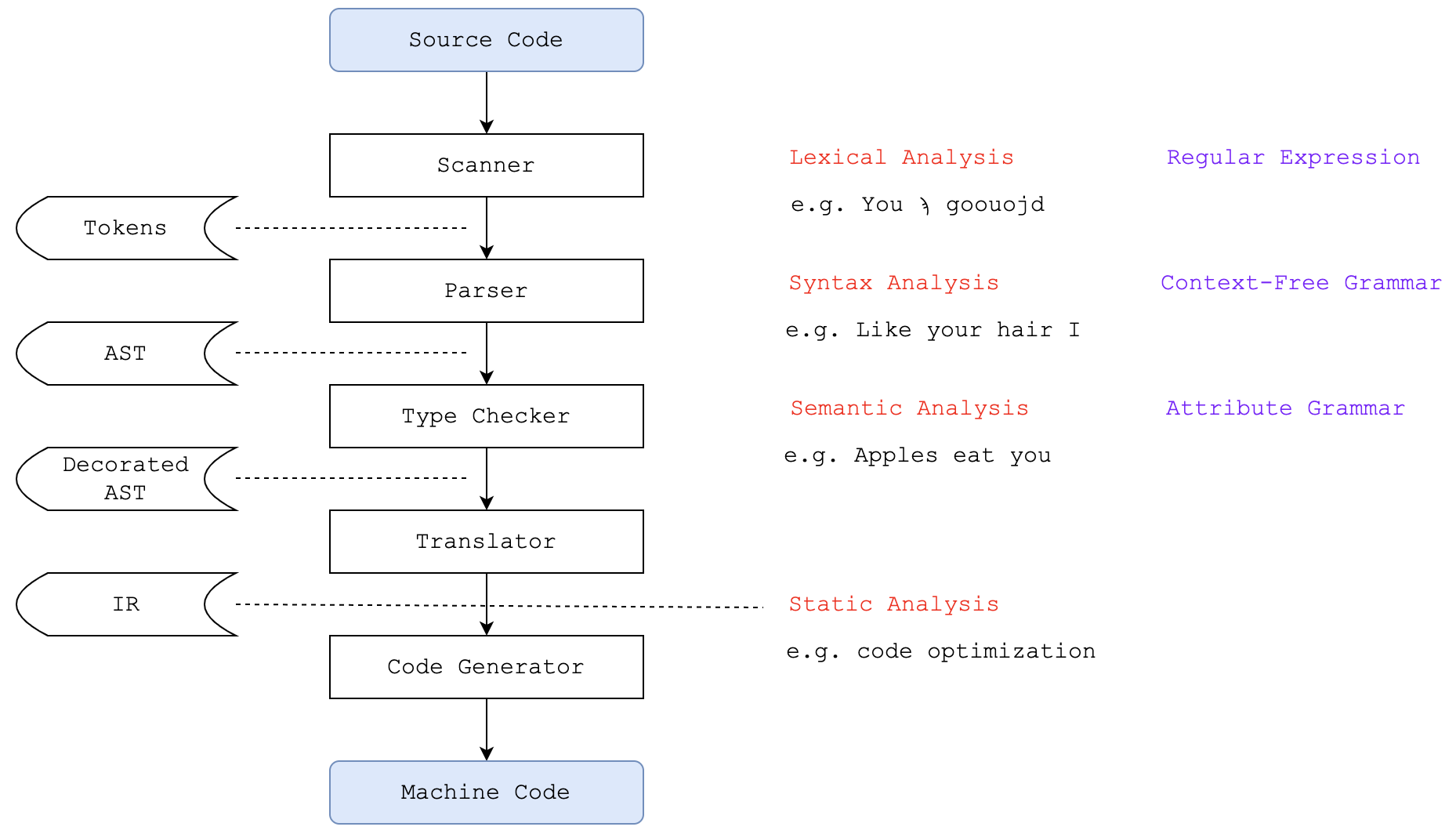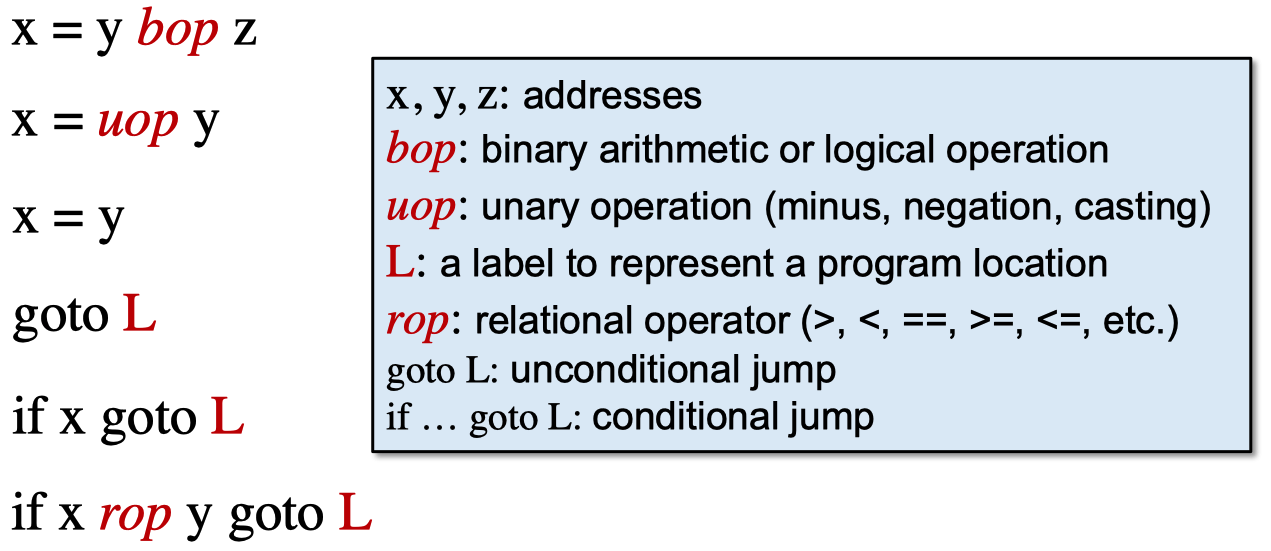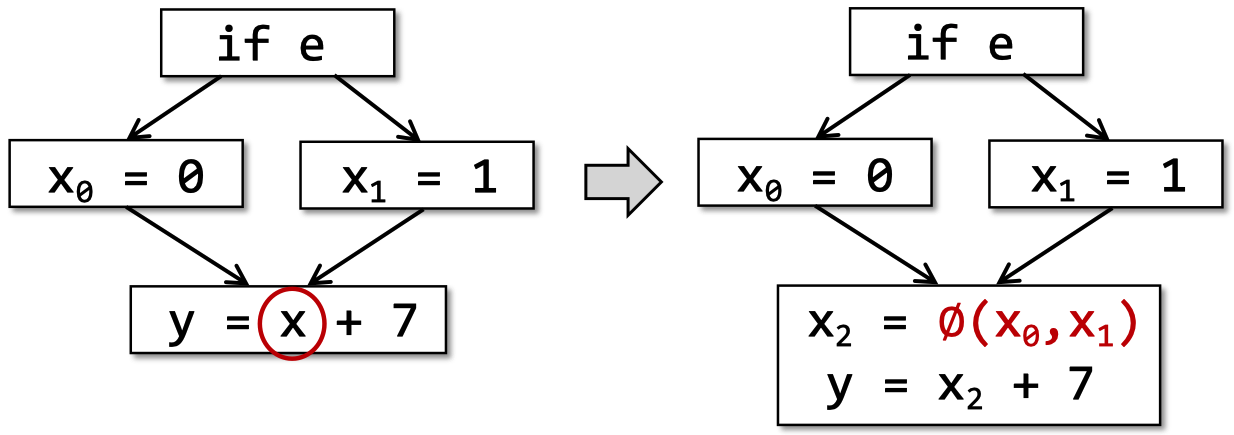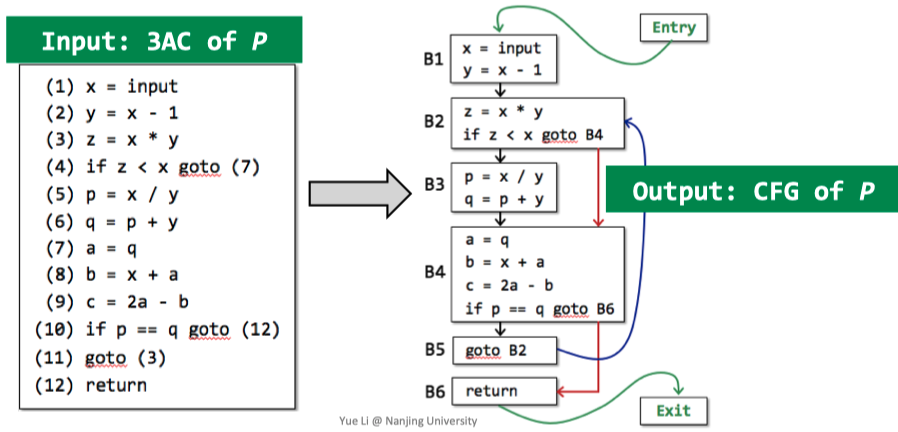## Compilers and Static Analyzers## AST vs. IR

do i = i + 1; while(a[i] < v);


graph TD; A(do-while)-->B1(body); A-->B2(<); B1-->C1(assign); B2-->C2("[ ]"); B2-->C3(v); C1-->D1("i"); C1-->D2("+"); C2-->D3(a); C2-->D4(i); D2-->E1("i"); D2-->E2("1");

1: i = i + 1
2: t1 = a [ i ]
3: if t1 < v goto 1


AST IR

- 通常用作静态分析的基础

IR常用的表示形式是三地址码（3-Address Code，简称3AC）。它的一个要求是，在一个指令的右边至多只有一个操作符。例如，对于c = a + b + 3这样的语句，3AC需要引入一个中间变量来将其变成两个指令：t1 = a + bc = t1 + 3## 3AC in Real Static Analyzer: Soot

javac DoWhileLoop3AC.java
java -cp sootclasses-trunk-jar-with-dependencies.jar soot.Main -cp . -pp -f jimple DoWhileLoop3AC


Do-While Loop

package nju.sa.examples;

public class DoWhileLoop3AC {
public static void main(String[] args) {
int[] arr = new int;
int i = 0;
do {
i = i + 1;
} while (arr[i] < 10);
}
}


Soot生成的Jimple文件（摘录了main函数部分）：

public static void main(java.lang.String[])
{
int[] r0;
int $i0, i1; java.lang.String[] r1; r1 := @parameter0: java.lang.String[]; r0 = newarray (int); i1 = 0; label1: i1 = i1 + 1;$i0 = r0[i1];
if $i0 < 10 goto label1; return; }  Method Call 示例代码： package nju.sa.examples; public class MethodCall3AC { String foo(String para1, String para2) { return para1 + " " + para2; } public static void main (String[] args) { MethodCall3AC mc = new MethodCall3AC(); String result = mc.foo("hello", "world"); } }  Soot生成的Jimple文件： foo函数： java.lang.String foo(java.lang.String, java.lang.String) { java.lang.StringBuilder$r0, $r2,$r3, $r5; java.lang.String r1, r4,$r6;
MethodCall3AC r7;
r7 := @this: MethodCall3AC;
r1 := @parameter0: java.lang.String;
r4 := @parameter1: java.lang.String;
$r0 = new java.lang.StringBuilder; specialinvoke$r0.<java.lang.StringBuilder: void <init>()>();
$r2 = virtualinvoke$r0.<java.lang.StringBuilder: java.lang.StringBuilder append(java.lang.String)>(r1);
$r3 = virtualinvoke$r2.<java.lang.StringBuilder: java.lang.StringBuilder append(java.lang.String)>(" ");
$r5 = virtualinvoke$r3.<java.lang.StringBuilder: java.lang.StringBuilder append(java.lang.String)>(r4);
$r6 = virtualinvoke$r5.<java.lang.StringBuilder: java.lang.String toString()>();
return $r6; }  main函数： public static void main(java.lang.String[]) { nju.sa.examples.MethodCall3AC$r0;
java.lang.String[] r3;
r3 := @parameter0: java.lang.String[];
$r0 = new nju.sa.examples.MethodCall3AC; specialinvoke$r0.<nju.sa.examples.MethodCall3AC: void <init>()>();
virtualinvoke $r0.<nju.sa.examples.MethodCall3AC: java.lang.String foo(java.lang.String,java.lang.String)>("hello", "world"); return; }  Class 示例代码： package nju.sa.examples; public class Class3AC { public static final double pi = 3.14; public static void main(String[] args) { } }  完整的Jimple文件： public class Class3AC extends java.lang.Object { public static final double pi; public void <init>() { Class3AC r0; r0 := @this: Class3AC; specialinvoke r0.<java.lang.Object: void <init>()>(); return; } public static void main(java.lang.String[]) { java.lang.String[] r0; r0 := @parameter0: java.lang.String[]; return; } public static void <clinit>() { <Class3AC: double pi> = 3.14; return; } }  ## Static Single Assignment (SSA) OK，接下来是静态单一赋值（SSA）这个概念。在3AC的基础上，SSA指的是，所有赋值操作的被赋值变量都需要有一个独特的名字。每个定义都会有一个新名字，这个新名字可以应用在后续的分析中，每个变量都有一个定义。下面是一个SSA的示例： 3AC: SSA: p = a + b p1 = a + b q = p - c q1 = p1 - c p = q * d p2 = q1 * d p = e - p p3 = e - p2 q = p + q q2 = p3 + q1  有时，某个变量可能受到条件分支影响，处于控制流汇聚的位置（control flow merges），此时就需要使用$\varphi$函数来处理，如下图所示：$\varphi(x_{0}, x_{1})$的值由控制流经过的条件分支决定。 关于SSA的讨论： 优点 缺点 控制流信息可以间接融合到独特变量名中，帮助简化分析 可能引入过多变量和$\varphi\$函数
Define-and-Use配对是明确清楚的 可能造成机器码生成效率低下

## Control Flow Analysis## Basic Blocks (BB)

BB指的是一个连续、最长的3AC序列，该序列具有以下特性：

• 控制流只能从该序列的起始指令进入。
• 控制流只能从该序列的最后一条指令退出。

1. 3AC序列中的第一条指令。
2. 所有有条件跳转或无条件跳转的所有目标指令。
3. 所有有条件跳转或无条件跳转后面的一条指令。

## Control Flow Graphs (CFG)

• CFG的节点均为BB。
• 从块A到块B之间有一个有向边，当且仅当“从A到B一个有条件或无条件跳转”，或“B是A后面的紧邻块且A最后一条指令不是无条件跳转”。
• 将原来3AC序列中的所有“跳转到某指令标签处”改为“跳转到某基本块处”。